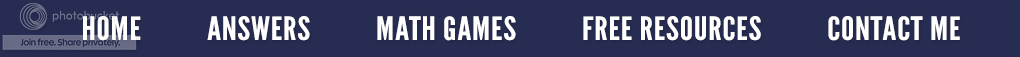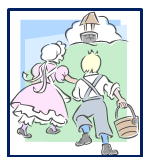### Jack and His Nines

My guest blogger today is Barbara.  Even though she is not a seller on Teachers Pay Teachers, we have been friends since 6th grade.  She originally lived in England, and I was her pen pal, but then she moved to my home town in Ohio where we became best friends.  She teaches at the University of North Carolina. The college has a drop in "math lab," which is a service to students who would like help with their math assignments. Barbara is one of five instructors who takes turns supervising the math lab and giving help.  She finds it to be a very rewarding job. The courses she mainly helps with are Statistics, Reality Math, Math for Teachers, Pre-Calculus through Calculus, a little Differential Equations, and occasionally some Physics.  I think you will find Barbara's article on the magical nines quite interesting.

****************************************************************************As the story goes, one day Jack and Jill were walking up the hill to fetch a pail of water. Jack said to Jill, “Did you bring your calculator?” Jill acknowledged that she never left home without it.

Jack said, “Okay, Jill, now think of a fairly large whole number and reverse the digits. Then subtract the smaller number from the larger.”  Using her calculator, Jill came up with an answer to which Jack replied, “Now choose one of the digits in the answer and remember it. Then tell me the other digits, in any order, and I will tell you the remaining digit.”

“Okeydokey,” said Jill, “the other digits are 7, 2, 5, and 1.” Jack revealed that the remaining digit was three.  “That’s right!” shouted Jill.  In fact, she was so surprised that she fell down and broke her crown.

?? How did Jack know Jill's number ??

The secret is in the properties of the number 9.  Did you know if you multiply ANY whole number by 9, the sum of the digits of the answer will also be a multiple of 9?

*A number is a multiple of 9 if its digits add up to 9 or a multiple of 9. If the answer is not a one digit number, continue to add the digits to eventually arrive at just a one digit answer of nine.  (This is sometimes referred to as finding the digital root.)

Example:  175,439,826 is divisible by 9 because when you add all the digits, you get 45. (1 + 7 + 5 + 4 + 4 + 9 + 8 + 2 + 6 = 45)  Adding the two digits of the answer, you get 9.  (4 + 5 = 9)   Nine is the digital root of 175,439,826 so this number is divisible by 9 as well as three since three is a factor of 9.

Example: 53,872,091 is NOT divisible by 9.  Adding the  eight digits you get the sum of 35. Adding the digits of the answer, 3 and 5, you get 8. This tells you that if you divide the original number by 9, you will get an answer with a remainder of 8.

Another interesting property of 9 is that if you choose any whole number, reverse its digits, and subtract the smaller number from the larger, the answer is always a multiple of 9.

Example:  5,132 – 2,315 = 2,817, which is divisible by 9.  (2 + 8 + 1 +7 = 18    1 + 8 = 9)

So Jack knew that when Jill subtracted her numbers, the answer was a multiple of 9. And when she told him the digits 7, 2, 5, and1, he knew that the remaining digit would have to be 3; so, that all the digits would add up to be a multiple of 9. (in this case, 18.)
Beware for there is one condition when this trick might not go as smoothly. If the digits already add up to be a multiple of 9, then the missing digit could be a 0 or a 9. You could then claim that your mental image is coming in fuzzy, and you can’t quite tell if it is a 0 or a 9.

Here is another math trick.  Ask someone to think of any three-digit number (with no repeating digits), reverse the digits, and subtract the smaller number from the larger. When that person has the answer, ask him/her to reverse the digits of the answer, and then add the answer and the reversed answer together.  You can then tell him/her that the final result is 1089. How do you know that? I will leave that for you to figure out! (That's problem solving at its best!)

P.S.  If you need help with the solution, check out the page above entitled Answers to Problems.